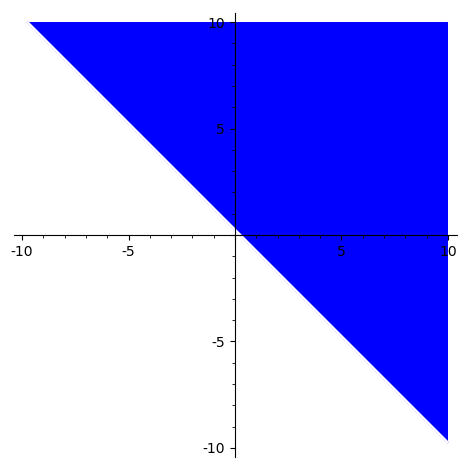# Plot all complex numbers, for which a predicate holds

How do I solve the following exercise in SageMath?

Outline (in the complex number plane) all numbers z in ℂ, for which abs(z+2)^2 > abs(z-2*I)^2+1 holds.

So, I figured the first step is to actually solve the inequation:

sage: sol=solve(abs(z+2)^2 > abs(z-2*I)^2+1, z) #0: solve_rat_ineq(ineq=(_SAGE_VAR_z+2)^2 > abs(_SAGE_VAR_z-2*%i)^2+1)
sage: sol
[[z < (2*I), (4*I + 4)*z + 7 > 0],
[z == (2*I), (8*I - 1) > 0],
[(2*I) < z, (4*I + 4)*z + 7 > 0]]


Okay, first, how do I interpret this solution? Every element in the list is a list of terms that must all hold? And of course, how can I now plot all those solutions?

edit retag close merge delete

It seems that teaching SageMath to do $|x+iy|^2=x^2+y^2$ is harder than doing it by hand.

Sort by » oldest newest most voted

We can also go the "blind way", using minimal mathematical effort and minimal code:

def f(x,y):
z = x+i*y
return abs(z+2)^2 > abs(z-2*i)^2 + 1

region_plot( f, xrange=(-6, 6), yrange=(-6, 6) )


And i've got the Launched png viewer for Graphics object consisting of 1 graphics primitive window. (The function to be plotted via region_plot delivers True or False. The graphic object highlights the True region.

moreLet me manually make the substitution $|x+iy|^2 = x^2 + y^2$ (it seems not easy to do in SageMath):

var('x,y')
ineqn = (x+2)^2 + y^2 > x^2 + (y-2)^2 + 1


Then you can plot immediately:

sage: region_plot(ineqn, (-10,10), (-10,10))You can also solve algebraically, using a slight workaround:

sage: ineqn.operator()((ineqn.lhs() - ineqn.rhs()).full_simplify(), 0)
4*x + 4*y - 1 > 0


Indeed, replacing ineqn by 4*x + 4*y - 1 > 0 produces the same picture.

You can also use the interface to QEPCAD to make a "cylindrical algebraic decomposition":

sage: qepcad(ineqn)
4 y + 4 x - 1 > 0


The output is a string, in QEPCAD syntax, which you can translate into SageMath by hand.

more